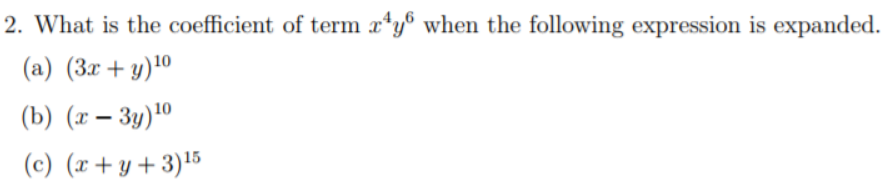# Thread: Discrete Math question

1. ##Discrete Math questionWhats the easiest way to approach questions like this?

Part (c) in particular.

Is the first answer 10c4 * 3^4 and last one 15c4 * 4c4?

Please can someone confirm?

2. ## Re: Discrete Math question

use the binomial theorem

$(a + b)^n = \sum \limits_{k=0}^n~\dbinom{n}{k}~a^k~b^{n-k}$

$(3x+y)^{10} = \sum \limits_{k=0}^{10}~\dbinom{10}{k}~(3x)^k~y^{10-k}$

we are clearly looking for the term that corresponds to $k=4$ which is

$\dbinom{10}{4}~3^4 x^4 y^6$

and thus the coefficient is

$\dbinom{10}{4}~3^4 = 17010$

use the same method on (b) and (c)

3. ## Re: Discrete Math questionOriginally Posted by romsekuse the binomial theorem

$(a + b)^n = \sum \limits_{k=0}^n~\dbinom{n}{k}~a^k~b^{n-k}$

$(3x+y)^{10} = \sum \limits_{k=0}^{10}~\dbinom{10}{k}~(3x)^k~y^{10-k}$

we are clearly looking for the term that corresponds to $k=4$ which is

$\dbinom{10}{4}~3^4 x^4 y^6$

and thus the coefficient is

$\dbinom{10}{4}~3^4 = 17010$

use the same method on (b) and (c)
Part (c) has 3 variables4. ## Re: Discrete Math questionOriginally Posted by inayatPart (c) has 3 variablesapply twice, adapt, improvise, overcome!

\begin{align*} &(x+y+3)^{15} = ((x+y)+3)^{15} = \\ \\ &\sum \limits_{k=0}^{15}~(x+y)^k~3^{15-k} = \\ \\ &\sum \limits_{k=0}^{15}~\left(\sum \limits_{j=0}^k~x^j~y^{k-j}\right)~3^{15-k} \end{align*}

5. ## Re: Discrete Math questionOriginally Posted by inayatPart (c) has 3 variablesOriginally Posted by inayatWhats the easiest way to approach questions like this?
Part (c) in particular.
last one 15c4 * 4c4? NO
In the expansion of ${(x + y + 3)^{15}}$
we have ${\dbinom{15}{5}}{(x + y)^{10}}{(3)^5}$
${\dbinom{15}{5}} \cdot {\dbinom{10}{4}}{y^{10 - 4}}{x^4}{(3)^5}$

You should get $153243090$ see HERE